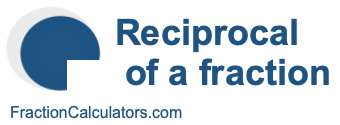Reciprocal of 6/8What is the reciprocal of 6/8? Here we will define reciprocal of 6/8, and show you how to calculate the reciprocal of 6/8 in fraction form and decimal form.

The reciprocal of 6/8 is a fraction or a number that when multiplied by 6/8 is equal to 1. To get the reciprocal of 6/8 based on that definition, we can make the following equation where "R" is the reciprocal of 6/8.

(6/8) × R = 1

When we solve for R, we get the answer to the reciprocal of 6/8 as a fraction as follows:

(6/8) × R = 1
R = 8/6
Reciprocal of 6/8 = 8/6

You can check that the answer is correct by confirming that 6/8 times the reciprocal of 6/8 is equal to 1, like this:

6/8 × 8/6 = 1

To find the decimal answer to the reciprocal of 6/8, you divide the numerator of the reciprocal by the demoninator of the reciprocal, like this:

8 ÷ 6 ≈ 1.333333333333
Reciprocal of 6/8 ≈ 1.333333333333

Tip: As you may have inferred from our tutorial above, you can quickly get the reciprocal of any fraction, such as 6/8, by switching the numerator and the denominator.

Reciprocal of a fraction
Do you need the reciprocal of another fraction? No problem! Please enter another fraction below.

Reciprocal of /

Reciprocal of 6/9
Here is the next fraction on our list that we have calculated the reciprocal of.